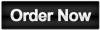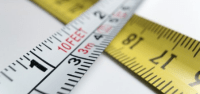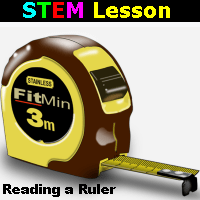This lesson introduces students to taking measurements with a standard ruler.

Lessons include:History of Measurement               Reading a Ruler                       Basic Measurements

Students learn the basics of measurement in inches, feet, years, halves, quarters, eighths and sixteenths.  Students also practice with fractional equivalents.

In miniature the first ten pages of the lesson are shown below:

230 RULER 10

At the completion of the lesson the students take a final quiz.  When students receive a score of 80%+ they will earn a badge on their account.  Actual scores are also emailed to the student.